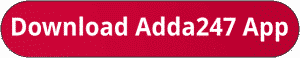Latest Banking jobs   »   sbi po quant

# Quantitative Aptitude Quiz For SBI PO/Clerk Prelims 2023 -21st October

Directions (1-5): In the given questions, two quantities are given, one as ‘Quantity I’ and another as ‘Quantity II’. You have to determine relationship between two quantities and choose the appropriate option:

Q1. ⅖th of P is equal to ¼ th of Q and 37 ½ % of (P + Q) is equal to 234.
Quantity I: What will be difference between P & Q.
Quantity II: 144.
(a) Quantity I > Quantity II
(b) Quantity I < Quantity II
(c) Quantity I ≥ Quantity II
(d) Quantity I = Quantity II or no relation
(e) Quantity I≤ Quantity II

Q2. A man invested Rs. P in a scheme offering 20% p.a. at CI compounding annually, at the end of two years interest received by him is Rs.7040.
Quantity I: Find value of P (in Rs.).
Quantity II: Rs.20000
(a) Quantity I < Quantity II
(b) Quantity I > Quantity II
(c) Quantity I = Quantity II or no relation
(d) Quantity I ≥ Quantity II
(e) Quantity I≤ Quantity II

Q3. Quantity I: If the radius and height of a cylinder is increased by 25% and 30%, then find out the percent change in its lateral surface area respectively.
Quantity II: 62.5%
(a) Quantity I = Quantity II or no relation
(b) Quantity I > Quantity II
(c) Quantity I < Quantity II
(d) Quantity I ≥ Quantity II
(e) Quantity I≤ Quantity II

Q4. A bag contains x blue balls and 5 pink balls. When two balls are drawn randomly from the bag, then the probability of getting 2 pink balls is 5/33.
Quantity I: Find x.
Quantity II: 6
(a) Quantity I = Quantity II or no relation
(b) Quantity I > Quantity II
(c) Quantity I < Quantity II
(d) Quantity I ≥ Quantity II
(e) Quantity I≤ Quantity II

Q5. Three years ago, the difference between the ages of Ajay and Vijay was 6 years. 10 years hence, ratio of ages of Ajay and Vijay will be 11 : 14 respectively.
Quantity I: Find the present age of Vijay.
Quantity II: 20 years.
(a) Quantity I = Quantity II or no relation
(b) Quantity I > Quantity II
(c) Quantity I < Quantity II
(d) Quantity I ≥ Quantity II
(e) Quantity I≤ Quantity II

Directions (6-10):- Each of the following questions is provided with 2 statements i.e. Statement I & Statement II. You have to study them and find which statement (s) is/are necessary to answer the question as per the instruction set given below.
(a) Only Statement I is sufficient
(b) Only Statement II is sufficient
(c) Neither Statement I nor Statement II is sufficient
(d) Either Statement I or Statement II is sufficient
(e) Both Statement I & Statement II together is sufficient

Q6. What is speed of car?
Statement I: Car can cover a distance of 100 km in 2 hour.
Statement II: Speed of bike is 20 kmph which is 40% of speed of car.

Q7. What is length of platform?
Statement I: a train covers the platform in 20 sec.
Statement II: the length of train is 50% of length of platform.

Q8. What is ratio of boys to girls in the class?
Statement I: total students in class are 100.
Statement II: boys are 20% more than girls.

Q9. What is present salary of Sanjay?
Statement I: Sanjay saves Rs. 500 which is 10% of his salary.
Statement II: Sanjay spends Rs. 2000 more than he saves.

Q10. What is present population?
Statement I: the population last year was 1000.
Statement II: population increases by 10% every year.

Solutions. .               .                                      .                       .                                         .

## FAQs

### What sections are included in the SBI PO or SBI Clerk Prelims Exam?

The selection process of SBI Clerk 2023 consists of Prelims, Mains and LPT.

#### Congratulations!Union Budget 2023-24: Free PDF